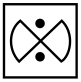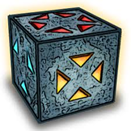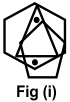# CREST Reasoning Olympiad Class 6 Sample Papers

REGISTER NOW

## Syllabus:

Section 1: Series Completion and Inserting the Missing Character, Analogy and Classification, Blood Relations, Coding - Decoding, Direction Sense Test, Logical Venn Diagram, Alpha Numeric Sequence Puzzle, Number, Ranking and Time Sequence Test, Logical Sequence of Words, Alphabet Test, Mathematical Operations, Analytical Reasoning, Mirror Images and Water Images, Embedded Figures, Figure Formation, Construction of Squares, Grouping of Identical Figures, Figure Matrix, Paper Folding and Paper Cutting, Cubes and Dice, Dot Situations.

Achievers Section: Higher Order Thinking Questions - Syllabus as per Section 1

 Q.1 Here two words are given which are related to each other in a particular manner and you have to find the word from the given alternatives which bears the exact same relationship to the third word, as the first two bears. 'Flower' is related to 'Petal' in the same way 'Book' is related to ______.
 Q.2 In the following series, replace the question mark (?) with a suitable option. 27, 32, 30, 35, 33, ?
 Q.3 How many 7's are there in the given series which are immediately preceded by 6 but not immediately followed by 8? 3 4 8 7 6 1 5 6 7 8 4 9 6 7 5
 Q.4 In each of following question, find the figure given in the answer choices, that can form the question figure.Q.5 Point D is towards the south of point C. Point B is to the east of point C. Point A is to the south of point B. Then in which direction is point D with respect to point A?
 Q.6 Which of the following will be the correct mirror image of the given figure, when the mirror is placed vertically to the right?Q.7 If in a certain code Language 'nik ka pa' means 'who are you', 'ka na ta da' means 'you may come here' and 'ho ta sa' means 'come and go', then what does 'nik' mean in that language?
 Q.8 Which of the following Venn diagram best represents the relationship among the given classes? Educated, employed, illiterate
 Q.9 In this question, relationship between different elements is shown in the statements. The statements are followed by two conclusions. Statement: H ≥ I = J > K ≤ LConclusions: (i) K < H (ii) L ≥ I
 Q.10 Select the figure which satisfies the same conditions of placement of the dots as in the figure (i).Sample PDF of CREST Reasoning Olympiad for Class 6: